# Glossary

Waldhausen

Web page

MathSciNet

Waldhausen: Algebraic K-theory of spaces. LNM 1126 (1985)

nLab: Waldhausen category

<]]>
Waldhausen S-construction
<]]>
Wall crossing
<]]>
Weak equivalence
<]]>
Weak generator

In a (pre-)triangulated category, a set of weak generators is a set of objects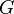such that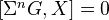for all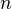andimplies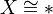.

<]]>
Weak homotopy equivalence
<]]>
Weak limit
<]]>
Weibel

Web page

MathSciNet

arXiv

See maybe also K-theory archive.

<]]>
Weight

arXiv:1003.0927 Weights in arithmetic geometry from arXiv Front: math.AG by Uwe Jannsen The concept of weights on the cohomology of algebraic varieties was initiated by fundamental ideas and work of A. Grothendieck and P. Deligne. It is deeply connected with the concept of motives and appeared first on the singular cohomology as the weights of (possibly mixed) Hodge structures and on the etale cohomology as the weights of eigenvalues of Frobenius. But weights also appear on algebraic fundamental groups and in p-adic Hodge theory, where they become only visible after applying the comparison functors of Fontaine. After rehearsing various versions of weights, we explain some more recent applications of weights, e.g., to Hasse principles and the computation of motivic cohomology, and discuss some open questions.

http://mathoverflow.net/questions/110179/weights-for-etale-cohomology-why-does-delignes-definition-work

Warning: I think that modular weight is different (perhaps plus/minus one) from geometric/motivic weight.

See David Hebert, on arxiv and maybe elsewhere, for weight structures on Beilinson motives. See also work of Bondarko.

http://mathoverflow.net/questions/31223/in-what-setting-does-one-usually-define-mixed-sheaves-and-weights-for-them

Check Deligne ICM1974 talk!

What is the weight spectral sequence? And what does "for weight reasons" mean, in spectral sequence arguments and elsewhere?

Andrzej Weber: Topological properties of the weight filtration (talk). Related to homology of complex algebraic varieties, and to intersection homology. Main point, I think: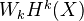and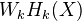are topological invariants.

http://mathoverflow.net/questions/54516/is-there-an-easy-proof-of-the-fact-that-the-intermediate-image-functor-respects-w

arXiv:1002.2651 Z[1/p]-motivic resolution of singularities from arXiv Front: math.KT by Mikhail V. Bondarko The main goal of this paper is to deduce (from a recent resolution of singularities result of Gabber) the following fact: (effective) Chow motives with $Z[1/p]$-coefficients over a perfect field $k$ of characteristic $p$ generate the category $DM^{eff}{gm}[1/p]$ (of effective geometric Voevodsky's motives with $Z[1/p]$-coefficients). It follows that $DM^{eff}{gm}[1/p]$ could be endowed with a Chow weight structure $w{Chow}$ whose heart is $Chow^{eff}[1/p]$ (weight structures were introduced in a preceding paper, where the existence of $w{Chow}$ for $DM^{eff}{gm}Q$ was also proved). As shown in previous papers, this statement immediately yields the existence of a conservative weight complex functor $DM^{eff}{gm}[1/p]\to K^b(Chow^{eff}[1/p])$ (which induces an isomorphism on $K0$-groups), as well as the existence of canonical and functorial (Chow)-weight spectral sequences and weight filtrations for any cohomology theory on $DM^{eff}{gm}[1/p]$. We also define a certain Chow t-structure for $DM_{-}^{eff}[1/p]$ and relate it with unramified cohomology. To this end we study birational motives and birational homotopy invariant sheaves with transfers.

arXiv:1102.4370 Weights on cohomology and invariants of singularities from arXiv Front: math.AG by Donu Arapura, Parsa Bakhtary, Jarosław Włodarczyk In this paper, we extract natural invariants of a singularity by using the Deligne weight filtration on the cohomology of an exceptional fibre of a resolution, and also on the intersection cohomology of the link. Our primary goal is to study and give natural bounds on the weights in terms of direct images of differential forms. These bounds can be made explicit for various standard classes such as rational, isolated normal Cohen-Macaulay and toroidal singularities, and lead to strong restrictions on the topology of these singularities. A secondary goal of this paper is to make the weight filtration, and related constructions, more widely accessible. So we have tried to make the presentation somewhat self contained. This is supersedes our earlier preprint arXiv:0902.4234.

<]]>
Weight filtration

http://mathoverflow.net/questions/117432/splitting-of-the-weight-filtration (there are also other MO questions on the weight filtration)

In the letter to Beilinson, Voevodsky formulates axioms for a homology theory. He considers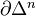as an (n-1)-dim sphere, write also S for the 1-dim sphere in this sense. Let Sch/k be the cat of separated schemes of finite type over a base k. Then a homological theory is a functor from Sch/k together with a family of natural isos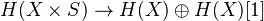. This functor should satisfy some conditions: Morally, homotopy invariance, MV exact triangle, an exact triangle for blowups, and transfer for flat finite morphisms. Get a 2-cat of homological theories over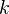. Examples: Algebraic K-th with rational coeffs, l-adic homology, Hodge homology ass to a complex embedding. Thm: There is an initial object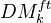in this cat, which we call the triang cat of eff mixed motives over k. Notion of reduced homological theory, and reduced motive of a scheme. Any motive in the above sense is of the form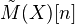, where we may assume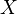affine and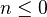. Tate object and comparison with K-theory. Bigger cat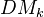which contains the previous as a full triang subcat, but admits a more explicit description rather than just the universal property. Can also be viewed as the closure of the previous, wrt direct sums and inductive limits. Need the h-topology, in particular coverings including surjective blowups, finite surjetive maps, etale coverings. Various filtrations on(homotopy canonical, geometrical, motivic canonical, weight). The weight filtr should be related to pure numerical motives.

<]]>
Weighted limit

Alex Shannon gave a talk at some point about something related I think.

<]]>
Weil

Collected papers, several volumes

<]]>
Weil conjectures

See Freitag-Kiehl, original articles of Dwork and Deligne, and lots of printed stuff in my office.

Kiehl and Weissauer: Weil conj, perverse sheaves, etc

Katz and Messing: Some consequences of the Riemann hypothesis for varieties over finite fields (1974)

http://mathoverflow.net/questions/72874/how-would-a-motivic-proof-of-the-riemann-hypothesis-over-finite-fields-go

http://mathoverflow.net/questions/33665/are-there-motivic-proofs-of-weil-conjectures-in-special-cases

http://sbseminar.wordpress.com/2010/05/03/the-weil-conjectures-the-approach-via-the-standard-conjectures/

http://sbseminar.wordpress.com/2010/04/12/the-weil-conjectures-and-the-problem-of-coefficients/

http://sbseminar.wordpress.com/2010/04/19/the-weil-conjectures-curves/

http://sbseminar.wordpress.com/2010/04/02/characteristic-zero-analogues-of-the-weil-conjectures-curves/

http://mathoverflow.net/questions/72834/what-exactly-does-the-weight-filtration-in-hodge-theory-have-to-do-with-the-weil

Some early writings:

• Chevalley, Bourbaki exp 3 and 9, for curves
• Exp 39, Delsarte
• Bourbaki exp 66 (Neron): Arithmetic on algebraic varieties

Irene had a book with some background.

The MathSciNet review of Deligne: La conjecture de Weil I, is very long and informative, with good historical references

<]]>
Weil pairing
<]]>
Weil-style algebraic geometry
<]]>
Weyl

Gesammelte Abhandlungen, 4 vols

<]]>

Mathematical works, 4 vols

<]]>
For two fibrant simplicial sets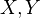, if a map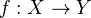is a weak equivalence, then it is a homotopy equivalence.